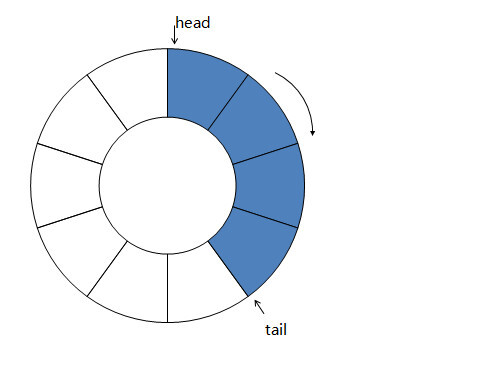# 手撕环形队列系列二：无锁实现高并发producer2 也向位置 0 写入数据，然后把 tail 增 1. tai 增加 1 的过程：

tail = tail + 1;

tail = 0 + 1 = 1;

cpu 的原子指令，是 cpu 指令级的细粒度锁，性能非常高，但设计起来复杂。

bool __sync_bool_compare_and_swap(type *ptr, type oldval， type newval);

// ring_queue.h#ifndef RING_QUEUE_H#define RING_QUEUE_Htypedef struct ring_queue_t {    char* pbuf;    int item_size;    int capacity;    volatile int write_flag;    volatile int read_flag;    volatile int head;    volatile int tail;    volatile int same_cycle;} ring_queue_t;int ring_queue_init(ring_queue_t* pqueue, int item_size, int capacity);void ring_queue_destroy(ring_queue_t* pqueue);int ring_queue_push(ring_queue_t* pqueue, void* pitem);int ring_queue_pop(ring_queue_t* pqueue, void* pitem);int ring_queue_is_empty(ring_queue_t* pqueue);int ring_queue_is_full(ring_queue_t* pqueue);#endif

// ring_queue.c#include "ring_queue.h"#include <stdlib.h>#include <string.h>#define CAS(ptr, old, new) __sync_bool_compare_and_swap(ptr, old, new)int ring_queue_init(ring_queue_t* pqueue, int item_size, int capacity) {    memset(pqueue, 0, sizeof(*pqueue));    pqueue->pbuf = (char*)malloc(item_size * capacity);    if (!pqueue->pbuf) {        return -1;    }    pqueue->item_size = item_size;    pqueue->capacity = capacity;    pqueue->same_cycle = 1;    return 0;}void ring_queue_destroy(ring_queue_t* pqueue) {    free(pqueue->pbuf);    memset(pqueue, 0, sizeof(*pqueue));}int ring_queue_push(ring_queue_t* pqueue, void* pitem) {    // try to set write flag    while (1) {        if (ring_queue_is_full(pqueue)) {            return -1;        }        if (CAS(&pqueue->write_flag, 0, 1)) {   // set write flag successfully            break;        }    }    // push data    memcpy(pqueue->pbuf + pqueue->tail * pqueue->item_size, pitem, pqueue->item_size);    pqueue->tail = (pqueue->tail + 1) % pqueue->capacity;    if (0 == pqueue->tail) {    // a new cycle        pqueue->same_cycle = 0;     // tail is not the same cycle with head    }    // reset write flag    CAS(&pqueue->write_flag, 1, 0);    return 0;}int ring_queue_pop(ring_queue_t* pqueue, void* pitem) {    // try to set read flag    while (1) {        if (ring_queue_is_empty(pqueue)) {            return -1;        }        if (CAS(&pqueue->read_flag, 0, 1)) {    // set read flag successfully            break;        }    }    // read data    memcpy(pitem, pqueue->pbuf + pqueue->head * pqueue->item_size, pqueue->item_size);    pqueue->head = (pqueue->head + 1) % pqueue->capacity;    if (0 == pqueue->head) {        pqueue->same_cycle = 1;     // head is now the same cycle with tail    }    // reset read flag    CAS(&pqueue->read_flag, 1, 0);    return 0;}int ring_queue_is_empty(ring_queue_t* pqueue) {    return (pqueue->head == pqueue->tail) && pqueue->same_cycle;}int ring_queue_is_full(ring_queue_t* pqueue) {    return (pqueue->head == pqueue->tail) && !pqueue->same_cycle;}## 评论